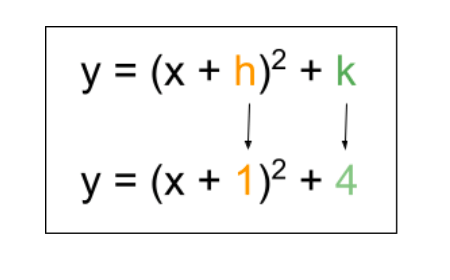# Vertex Form Calculator

Choose the equation form and enter the values of a, b, and c in the vertex form calculator to find vertex and y-intercept.

Vertex form

a(x - h)² + k## Vertex Form Calculator

The vertex form calculator is used to find the vertex and y-intercept for a parabola. You can find the vertex for a standard quadratic form and vertex form of a parabola.

## What is a vertex?

A vertex is the intersection point of the x and y coordinates of a parabola. It is the extremal point on its graph. It can be a minimum or maximum point.

The point is generally written as p(h,k). Here h is the x-coordinate and k is the y-coordinate.

## Vertex formula:

Vertex is calculated from two types of equations: standard and vertex form.

### For standard form (y = ax2 + bx + c):

h = -b/2a

k = c - b2/(4a)

(Alternatively, you can convert standard form into vertex form to identify the values.)

### For vertex form (y = a*(x-h)2 + k) :

Simply identify h and k

## How to calculate the vertex of a parabola?

### Example:

Find the vertex for the equation:

y = 3x2 + 2x + 5

Solution:

Step 1: Complete the square:

y = x2 + 2x + 5

y - 5 + 1 = x2 + 2x +1 (Adding 1 to the both sides)

y - 4 = (x + 1)2

y = (x + 1)2 + 4

Now it is in vertex form.

Step 2: Identify h and k: